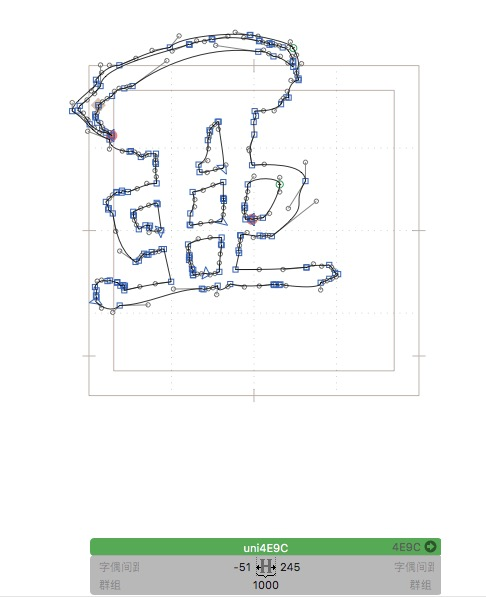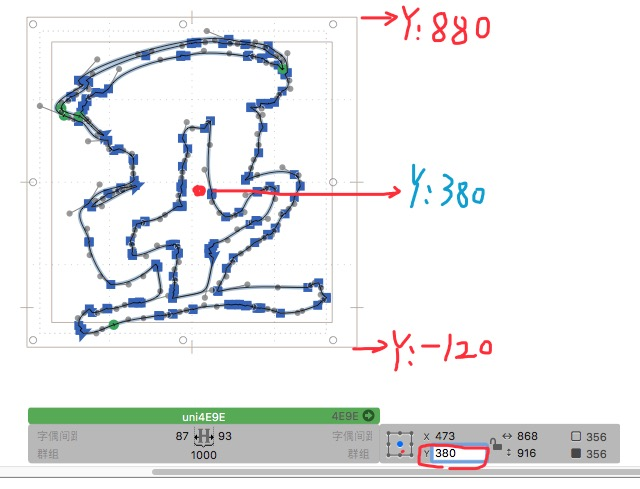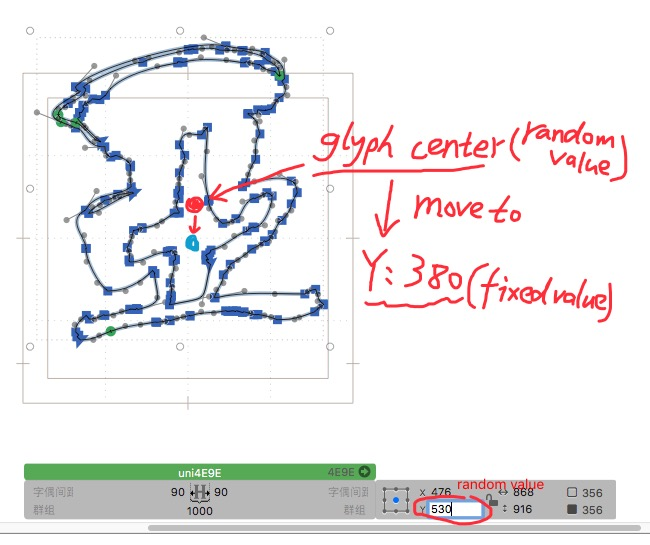# How to define the vertical width in Python？

Hi.I want to make the glyphs center in vertical height, I modified a python script,
I don’t know how to define vertical width.
How to define the vertical width in Python:sweat:
Or what other quick ways to do it.
I have a large number of glyphs that need to be centered.

import sys
import os
from GlyphsApp import *
import objc

def main():
Doc = Glyphs.currentDocument
Font = Doc.font
SelectedLayers = Doc.selectedLayers()
for Layer in SelectedLayers:
TSB = Layer.TSB
BSB = Layer.BSB
Vertical width = Layer.vertical width
newSB = (TSB + BSB) / 2.0
print TSB, BSB, newSB
Layer.TSB = newSB
Layer.vertical width = Vertical width

if name == ‘main’:
main()

``````Vertical width = Layer.vertical width
^
``````

SyntaxError: invalid syntax:slightly_frowning_face:The vertical width/TSB/BSB are used for vertical layout. If you like to center the glyph for horizontal layout, you just move the outlines. You can use `layer.applyTransform((1, 0, 0, 1, X, Y))`, The argument is an transformation matrix. The last two numbers are the X and Y offset.

I want to center vertically. The height of each glyph is different, I did not find a quick solution.How to define the vertical center point of the font？

layer = Glyphs.font.selectedLayers # current layer

yCenter = ?? # How should I write here，I didn’t find the definition value of this
shift = 380 - yCenter

layer.applyTransform([
1, # x scale factor
0.0, # x skew factor
0.0, # y skew factor
1, # y scale factor
0.0, # x position
shift # y position
])

``````from Foundation import NSMidY
bounds = Layer.bounds
centerY = NSMidY(bounds)
``````Great, Thank you very very much

I want to process multiple selected glyphs at once.
This can only be processed one at a time.
Can you help me?for myGlyph in Glyphs.font.glyphs:
layer = Glyphs.font.selectedLayers # current layer
from Foundation import NSMidY
bounds = Layer.bounds
centerY = NSMidY(bounds)

``````myAscender = Font.selectedFontMaster.ascender
myDescender = Font.selectedFontMaster.descender
mycenterY = (myAscender + myDescender) / 2.0

shift = mycenterY - centerY

Layer.applyTransform([
1, # x scale factor
0.0, # x skew factor
0.0, # y skew factor
1, # y scale factor
0.0, # x position
shift # y position
])
``````